Vibroengineering PROCEDIA

Published: 28 November 2019

# Vibration analysis of cantilever beam in time domain and frequency domain using Arduino platform

Chetan Reddy1
Sucheth Shenoy2
Ramesh S. Sharma3
1, 2, 3Department of Mechanical Engineering, Rashtreeya Vidyalaya College of Engineering, Bangalore, 560 059, Karnataka, India
Corresponding Author:
Chetan Reddy
Views 1178

#### Abstract

In this paper, analysis is made in the field of mechanical vibrations using Arduino and MATLAB code. Test was conducted on a cantilever beam to extract the first three natural frequencies. Two specimens made of Aluminium and Mild Steel were considered for the analysis. The frequencies were first determined using experimental setup utilizing Arduino and MATLAB, later verified with the help of two methods: (1) Traditional “strike method” using 8-channel FFT analyser with Data Acquisition System (2) Analytical solutions available in Robert D Blevins for idealized continuous beam model. The results obtained for both specimens through these methods were compared with Arduino MATLAB code and found to be in very good agreement. Hence, in this work an attempt is made with the use of Arduino to extract few frequencies for simple structures which is less expensive, fast and provides reasonably good results and can be a substitute for FFT analyser, which is very expensive and time consuming.

## 1. Introduction

There are number of software available for the analysis of mechanical vibrations, though the use of FFT Analyser Data Acquisition system with processing software known as DEWESOFT has been extensive. The software, although accurate and favoured, is very expensive hence an attempt is made to introduce the use of Arduino along with MATLAB to gain the same accurate results in a more economical way [1, 2].

Arduino Uno is an open source microcontroller which is used widely owing to its flexibility and inexpensiveness . It is built around the ATMEGA328P microchip and is equipped with 14 digital and 6 analog input/output pins. The programs are written in the Arduino IDE, which is a cross platform application. The transfer of data between the computer and the Arduino is via the USB. A dataset consisting of the acceleration values and their corresponding timestamps are produced as the output by the Arduino [4, 5].

MATLAB software is used in this analysis to mainly represent the data obtained from the Arduino. Use of MATLAB will enable plotting the PSD curves that will give the natural frequencies for the structure on which the experiment is being performed.

The objective of this work is to replace the expensive FFT Analyser Data Acquisition system with the significantly cost effective Arduino in extracting few fundamental frequencies of the specimen . For this, sensor has been used, which gives the real time data. This data is then used by MATLAB to provide the natural frequencies, which is then compared with the analytical as well as FFT analyser results. The use of sensors and microcontrollers to perform the experiment has made it very economical.

## 2. Experimental and analytical methods

In this work, the main focus is to extract the first three modes of specimens made of Mild Steel and 6061 Aluminium under cantilever condition [6, 7]. This is carried out using three different methods. In the first method, 8 channel FFT Analyser with DAQ system is used and further processed using DEWESOFT software. Secondly, use of Arduino to record the accelerometer data and further analysed and represented using MATLAB. Finally, validating the results of the above two methods using the analytical solution available in the literature.

### 2.1. Analytical method

First the three modes for Mild steel and 6061 Aluminium specimens were determined analytically . The material properties and the material dimensions were set before proceeding with the determination of frequencies. Using the boundary conditions and solving the partial differential equation the Eigen values were found which represent the natural frequencies of the structure [9, 10].

### 2.2. Experimental modal test

The natural frequencies through modal testing were extracted using 8-channel FFT analyser. In this method, a piezoelectric accelerometer was placed on the free end of the beam and an impulse hammer was used to impact the specimen at different locations marked on the specimen. The response obtained through accelerometer was fed to FFT Analyzer and processing was carried out using DEWESOFT software which finally provided Frequency. The experiment was repeated for both the specimens and average of all the frequencies obtained from several trials was considered. The modal test setup is shown in Fig. 2.

Fig. 1Experimental setup for Arduino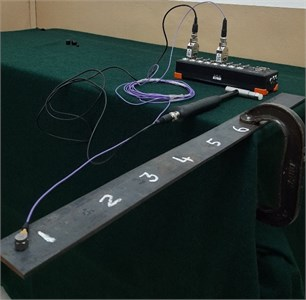Fig. 2Experimental setup for FFT analyser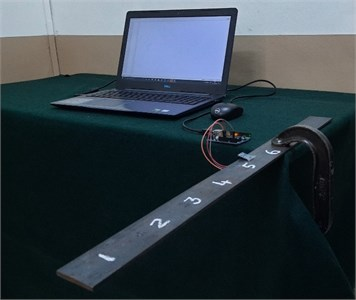### 2.3. Arduino with MATLAB method

Finally, in the third method of determining the natural frequencies, an ADXL-335 analog accelerometer was used to sense the acceleration of the specimen [11, 12] shown in Fig. 1. The accelerometer was fixed on the top surface of the specimen at its free end. An Arduino Uno was used to read the acceleration data at a programmed sample rate and log the data alongside its corresponding time stamps onto the serial monitor . The data from the serial monitor was then copied into a spreadsheet. The graphs were plotted using the readings that were obtained from the spreadsheet using MATLAB. Analysis of the graph was carried out . In MATLAB the inbuilt function called ‘pwelch’ was used to directly obtain the PSD curves .

## 3. Results and discussions

### 3.1. Aluminium specimen

Table for aluminium fixed-free beam used to compare the results obtained from analytical, Arduino and FFT analyser.

Fig. 3FRF graph for aluminium using the FFT analyzer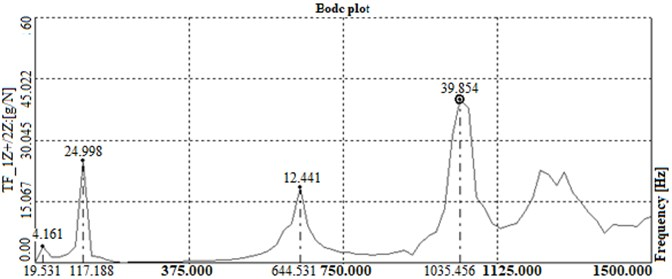Fig. 4PSD graph for aluminium using the MATLAB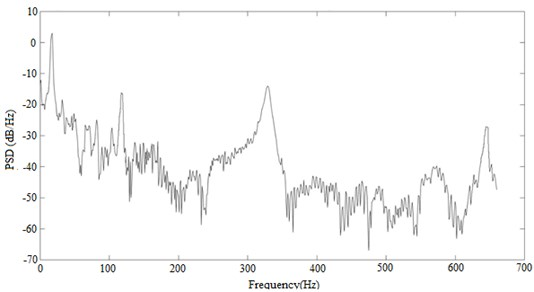Table 1Frequencies obtained for aluminium

 Sl. No. Analytical Arduino FRF 1 19.45 18.58 19.53 2 121.13 118.4 117.18 3 341.41 328.4 - 4 658.89 639.45 644.53

### 3.2. Mild steel specimen

Table for Mild steel fixed-free beam frequencies for results obtained from analytical, Arduino and FFT analyser.

Table 2Frequencies obtained for mild steel

 Sl. No. Analytical Arduino FRF 1 23.34 21.5 19.53 2 140.13 136.1 117 3 203.56 198.34 195.31 4 409.65 394.1 410

The results obtained through Modal Test and analytical solution are compared with the results obtained from Arduino with MATLAB Code. It is evident that the deviation between the three results obtained are reasonably acceptable. This in essence means that for obtaining the natural frequency for any given material, using Arduino along with MATLAB is a much cost effective way as it provides the results with very accessible and affordable sensors and microcontrollers [4, 16]. The natural frequencies obtained from the Analytical solution are then further placed alongside and compared with experimental values. It is seen that the values obtained experimentally do not deviate much from the analytical solution and the error incurred in the process is well below the acceptable values, i.e. < 8 % .

Fig. 5FRF graph for mild steel using the FFT analyzer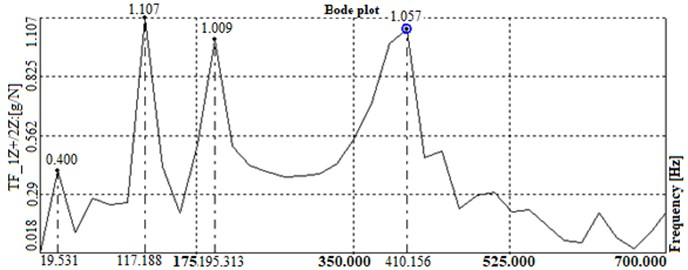Fig. 6PSD graph for mild steel using MATLAB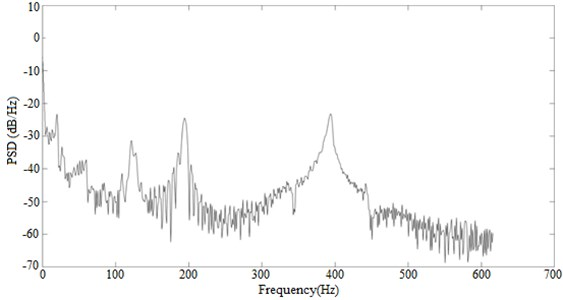## 4. Conclusions

After the detailed experimentation, it can be safely concluded that Arduino can be a cost effective replacement or substitute for the expensive FFT analyser with processing software currently available in the market . By replacing with Arduino, the error produced is acceptable (< 8 %) and therefore is a viable option for any organization and institutions that are looking for affordable means. Further, the setup needed for Arduino is less tedious compared to FFT Analyzer which is more expensive and time consuming. Using Arduino also gives the chance for inter-disciplinary collaboration, since the sensors used will facilitate computer software to read and interpret mechanical systems .

#### Acknowledgements

The authors thankfully acknowledge the Principal, Prof. & Head, Department of Mechanical Engineering, and the Management of R V College of Engineering, Bangalore for their constant support and encouragement extended during the course of this project.